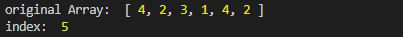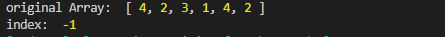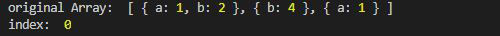# Lodash _.findLastIndex() Function

Lodash is a JavaScript library that works on the top of underscore.js. Lodash helps in working with arrays, strings, objects, numbers, etc.
The _.findLastIndex() function is used to find the element from the right of the array. Therefore giving the index of the last occurrence of the element in the array.

Syntax:

```findLastIndex(array, [predicate=_.identity], fromIndex);
```

Parameter:

• array: It is the original array.
• predicate: It is the function that iterates over each element.
• fromIndex: It is the index after which the searching starts. If from Index is not given then by default it is n-1 where n is the length of the array.

Return Value: It returns the index of the element if found else -1 is returned.

Note: Install lodash module by using command `npm install lodash` before using the code given below.

Example 1:

 `// Requiring the lodash library ` `const _ = require(``"lodash"``); ` ` `  `// Original array ` `let array1 = [4, 2, 3, 1, 4, 2] ` ` `  `// Using lodash.findLastIndex ` `let index = _.findLastIndex(array1, (e) => { ` `    ``return` `e == 2; ` `}); ` ` `  `// Original Array ` `console.log(``"original Array: "``, array1) ` ` `  `// Printing the index ` `console.log(``"index: "``, index) `

Output:Example 2: When an element is present in the array but the output is -1 because it is present after the from the index. Here fromIndex is 2.

 `// Requiring the lodash library ` `const _ = require(``"lodash"``); ` ` `  `// Original array ` `let array1 = [4, 2, 3, 1, 4, 2] ` ` `  `// Using lodash.findLastIndex ` `let index = _.findLastIndex(array1, (e) => { ` `    ``return` `e == 1; ` `}, 2); ` ` `  `// Original Array ` `console.log(``"original Array: "``, array1) ` ` `  `// Printing the index ` `console.log(``"index: "``, index) `

Output:Example 3: When an array of objects is given.

 `// Requiring the lodash library ` `const _ = require(``"lodash"``); ` ` `  `// Original array ` `let array1 = [ ` `    ``{ ``"a"``: 1, ``"b"``: 2 },  ` `    ``{ ``"b"``: 4 },  ` `    ``{ ``"a"``: 1 } ` `] ` ` `  `// Using lodash.findLastIndex ` `let index = lodash.findLastIndex(array1, (e) => { ` `    ``return` `e.b == 2; ` `}, 2); ` ` `  `// Original Array ` `console.log(``"original Array: "``, array1) ` ` `  `// Printing the index ` `console.log(``"index: "``, index) `

Output:My Personal Notes arrow_drop_upCheck out this Author's contributed articles.

If you like GeeksforGeeks and would like to contribute, you can also write an article using contribute.geeksforgeeks.org or mail your article to contribute@geeksforgeeks.org. See your article appearing on the GeeksforGeeks main page and help other Geeks.

Please Improve this article if you find anything incorrect by clicking on the "Improve Article" button below.

Article Tags :

Be the First to upvote.

Please write to us at contribute@geeksforgeeks.org to report any issue with the above content.